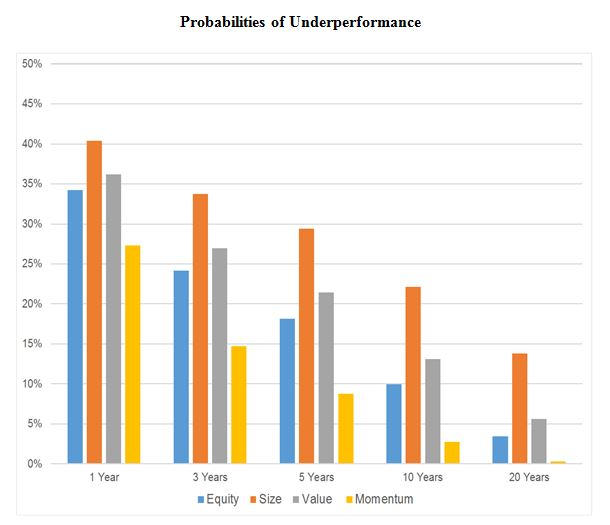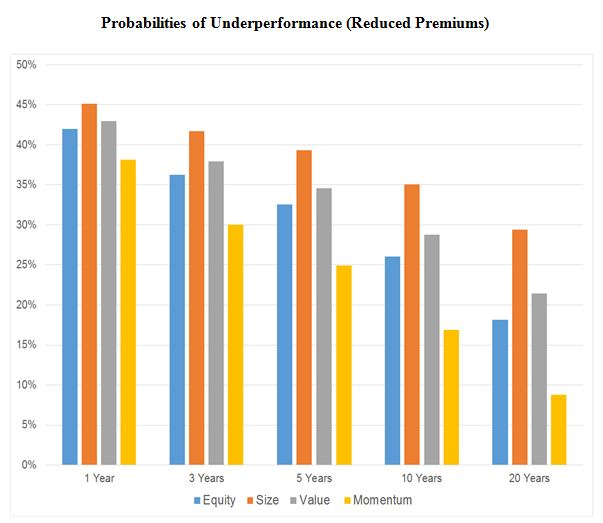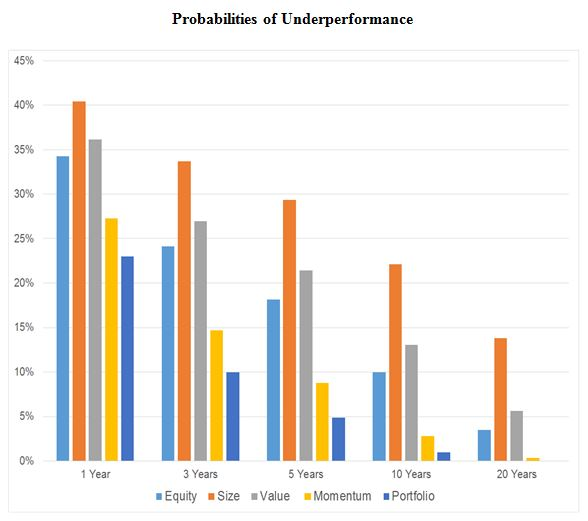# Best Of 2016: Swedroe On The Mystery Of Vanishing Premiums

February 16, 2016The graph above shows that the probabilities of underperformance are significant and positive all the way out to five years. And even beyond five years, three of the four premiums have a greater than 10% chance of underperforming over a 10-year horizon.

Kizer then looked at the same data, but cut his estimates regarding the size of each premium in half. He kept the volatilities the same. This is reasonable to look at, since one could argue all of these premiums may be lower in the future than they have been in the past due to post-publication effects (and various other considerations, like trading costs and investment management expenses). His results are shown in the graph below.Here there is a significant jump in the probabilities of underperformance. Out to five years, there are now roughly 25%-plus probabilities of underperformance. At the 10-year horizon, the probabilities are all in excess of 15%. While some could certainly argue that a 50% reduction in each premium is severe, this nevertheless illustrates how sensitive the probabilities are to the assumed size of each premium.

Role Of Diversification

Finally, Kizer examined the importance of diversification in helping to limit the probability of underperformance. As a rough illustration, he built a portfolio allocated 25% to each of the four premiums, then replicated the probability of underperformance from his first graph (keeping the size of each premium at its historical value).As you can observe in the graph above, diversification across each of the four factors greatly reduces the probability of underperformance, which perhaps is not surprising given the low correlation across each of these factor premiums.

The evidence presented does make a strong case for diversifying across the various investment factors that have been shown to explain the vast majority of the differences in returns of diversified portfolios. Each of these factors is supported by evidence that is: